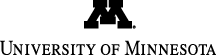CSci 5302 - Analysis of Numerical Algorithms
index.php

# CSci 5302 - Updated Assignment Plan

```
Total points during entire semester

100%  ....  total points

Items so far,

* 15%    1  midterm exam [15% of final grade, under original plan]
* 30%    4  homeworks [7.5% each assuming there would have been 6 HWs in all]
* 06%    6  quizzes (classroom exercises) [1% each]

51%  ....  total points so far

49%  ....  total remaining points

Distribution of remaining points under original plan:

* 30%    2  midterm exam [15% of final grade, under original plan]
* 15%    2  homeworks [7.5% each assuming there would have been 6 HWs in all]
* 04%    4  quizzes (classroom exercises) [1% each]

Distribution of remaining points under revised plan:

* 24%    2  "enhanced" homeworks or "take-home exams" [12% each]
* 20%    2  homeworks [10% each]
* 05%    5  quizzes (like the classroom exercises) [1% each]

```

The "enhanced" homeworks will have the same ground rules as regular homeworks:

• Do them by yourself. The work submitted must represent your own solution to the questions asked;
• You can use your notes, the book, your computer and calculator, reference materials from the web, etc.;
• The answers will be submitted through Canvas just like regular homeworks.

But the enhanced homeworks will differ from regular homeworks in the following way:

• They will be due the day after being assigned. The exact due date and time will be specified when assigned.
• The enhanced homeworks will be assigned on the days previously announced for the in-class midterms.
• There will be no more in-class midterms.